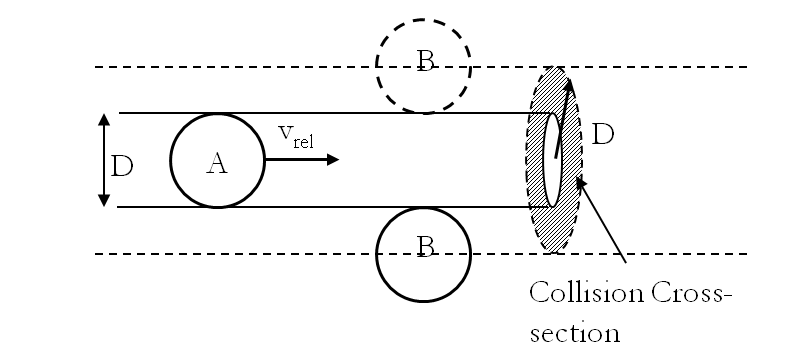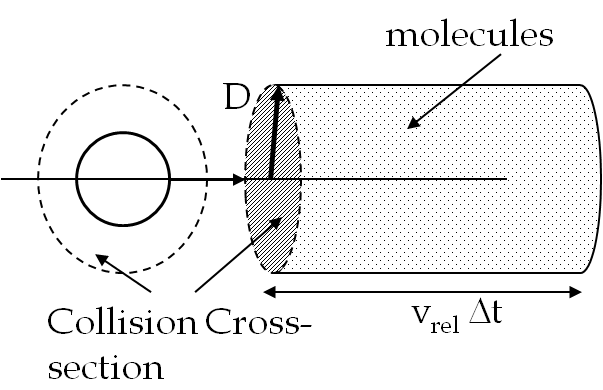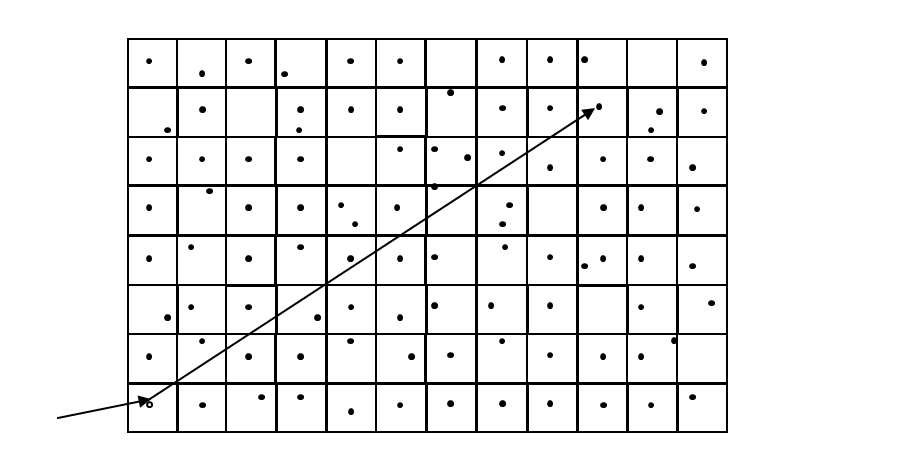## Section27.3Mean Free Path

Unlike ideal gases the molecules of real gases interact with each other and collide which changes their momenta. The average distance traveled by a molecule between collisions is called the mean free path. To get a useful estimate of the mean free path, we model molecules as spheres of diameter $d\text{,}$ and look at the collision cross-section between two molecules $A$ and $B$ as shown in Figure 27.3.1, which is drawn in the rest frame of $B\text{.}$ Let $v_{rel}$ be average relative speed between two molecules.Figure 27.3.1. Collision of molecules $A$ and $B$ in the rest frame of $B\text{.}$ Molecules $A$ and $B$ will collide if they are within the circle of radius $D\text{,}$ which is taken to be the diameter of one molecule.

Molecule A will collide with molecule B if latter's center is within a distance $D$ of the center of the molecule $A$ as shown in Fig. \ref{fig:mean-free-path}. Therefore the cross-section for collision is equal to $\pi D^2\text{.}$

The number of collisions suffered by a molecule will depend on the density of molecules and their relative speed. Although after each collision the direction of motion of a molecule will change, but in each direction it is the same cross-sectional area. Therefore the number of collisions in some duration $\Delta t$ will equal the number of molecules in the volume of a cylinder of the cross-sectional area $\pi D^2$ and length $v_{rel} \Delta t\text{,}$ the distance traveled by the molecule shown as A (Figure 27.3.2).Figure 27.3.2. Molecules in the enclosed cylinder will collide with a selected molecule
\begin{align*} \amp \text{Number of collisions in } \Delta t = \dfrac{N}{V}\left(\pi D^2 v_{rel}\Delta t \right)\\ \amp \text{Distance traveled in } \Delta t = v_\text{ave} \Delta t \end{align*}

where $v_\text{ave}$ is the average speed of the molecule, $N$ is the total number of molecules occupying volume $V\text{.}$ It can be shown that the average speed is related to the average relative speed as follows.

\begin{equation*} v_{rel} = \dfrac{ v_\text{ave} }{\sqrt{2}} \end{equation*}

Hence, mean free path $\lambda$ is

\begin{equation*} \text{Mean free path, }\lambda = \dfrac{\text{Distance traveled}}{\text{Number of Collisions}} = \dfrac{1}{\sqrt{2}\ \pi D^2}\dfrac{V}{N}. \end{equation*}

Because most real gases at high temperature and low density behave as ideal gas, sometimes we can use the ideal gas law even for real gases. In that case we can replace $V/N$ and obtain mean free path in terms of temperature and pressure.

\begin{align*} \text{Approximately Ideal}\text{ Gas} \amp \text{ Behavior:} \lambda = \dfrac{k_B}{\sqrt{2}\ \pi D^2}\dfrac{T}{p}. \end{align*}

You might call this “ideal gas mean free path”. In a strict sense, a true ideal gas will have $\lambda = \infty\text{,}$ since the molecules of an ideal gas do not interact with each other. This formula is a mixture of ideas from the real gas and the ideal gas and the final mean free path formula is often called the “ideal gas mean free path”.

Find the ideal mean free path for a gas at standard temperature and pressure (STP), i.e. a temperature $T = 300\text{K}$ and a pressure $p = 1\text{ atm}\text{,}$ for a molecule of size $0.4\text{ nm}$ and compare it to the average distance between molecules.

Hint

Use formulas.

$\lambda = 52\ \text{nm},\ d=3.3\ \text{nm}$

Solution

We use the formula for mean free path for an ideal gas given in terms of $T$ and $p$ to obtain

\begin{equation*} \lambda_{\text{ideal}} = \frac{k_B}{\sqrt{2}\ \pi D^2}\frac{T}{p} = 52\ \text{nm}. \end{equation*}

Average separation between molecules can be obtained from the average space occupied by one molecule. If the average space occupied by one molecule as a sphere of radius $R\text{,}$ The average separation between molecules $d_\text{ave}$ will be $2R\text{.}$

\begin{equation*} d_\text{ave} = 2\left(\frac{3k_B T}{4\pi p} \right)^{1/3} = 3.3\ \text{nm}. \end{equation*}

Thus, at standard temperature and pressure (STP) mean free path $\lambda >> \text{ average distance between molecules}$ as shown in Figure 27.3.4.Figure 27.3.4. Molecular size, the separation of molecules and the mean free path illustrated approximately to scale in an ideal gas at 300 K and 1 atm.

Find the (a) mean free path, (b) mean free time, and (c) inter-molecular distance in a container of volume 5 L that contains 2 grams of Helium gas at $25^{\circ}$C and 1 atmosphere, assuming the diameter of each Helium molecule to be $1.0 \ \unicode{x212B}\text{.}$ Mean free time is the average time a molecule spends in-between collisions. ($1.0 \ \unicode{x212B}= 0.1\ \text{nm}$).

Hint

Use defining formulas.

(a) $914\text{ nm}\text{,}$ (b) $0.725\text{ ns}\text{,}$ (c) $2.55\ \text{nm}\text{.}$

Solution 1 (a)

(a) We us the formula for the mean free path

\begin{equation*} \lambda = \frac{k_B}{\sqrt{2}\pi D^2}\frac{T}{p}, \end{equation*}

where $N_A$ is the Avogadro number. The given system has

\begin{align*} \amp p = 1.013\times 10^5\ \text{Pa}\\ \amp T = 25+273 = 298\ \text{K}\\ \amp D = 1\times 10^{-10}\ \text{m}\\ \amp k_B = 1.38\times 10^{-23}\ \text{J/K}. \end{align*}

The mean free path equals $914\text{ nm}\text{.}$

Solution 2 (b)

(b) To find the mean free time we will use the average speed of molecules.

\begin{equation*} v_{ave} = \sqrt{8 k_b T/\pi m} = 1260\ \text{m/s}. \end{equation*}

Therefore, the mean free time will be

\begin{equation*} \tau = \frac{\lambda}{v_{ave}} = \frac{914 \times 10^{-9}\ \text{m}}{1260\ \text{m/s}} = 0.725 \text{ ns}. \end{equation*}
Solution 3 (c)

(c) From the number of molecules spread over the volume of the container we can estimate the average inter-molecular distance. Let $l$ be the average distance between molecules, $N$ the number of molecules, and $V$ the volume of the container. Then we can assume dividing up the volume in little cubes of side $l$ and place one molecule in each cube. This will give

\begin{equation*} N l^3 = V, \end{equation*}

Therefore,

\begin{equation*} l = (V/N)^{1/3}. \end{equation*}

Here

\begin{equation*} V = 5\ \text{L},\ \ N = \frac{2\ \text{g}}{4\ \text{g}/6.022\times 10^{23}\ \text{molecules}} = 3.011\times 10^{23}\ \text{molecules}. \end{equation*}

Therefore,

\begin{equation*} l = (5\times 10^{-3} \text{m}^3/3.011\times 10^{23})^{1/3} = 2.55\ \text{nm}. \end{equation*}

What will happen to the mean free path in Checkpoint 27.3.5 if we do the following:

(a) double the pressure while keeping the other quantities same?

(b) double the temperature while keeping the other quantities same?

(c) double the volume while keeping the other quantities same?

(d) double the amount of gas, i.e. mass, while keeping the other quantities same?

(e) double the mass and volume while keeping the other quantities same?

Hint

Think proportionality relations, e.g., for (a) $\lambda \sim 1/p\text{.}$

See the solutions.

Solution 1 (a)

(a) Since $\lambda \sim 1/p\text{,}$ doubling $p$ would halve $\lambda\text{.}$

Solution 2 (b)

(b) Since $\lambda \sim T\text{,}$ doubling the temperature in the Absolute scale $T$ would make $\lambda$ twice as large. This makes sense since the speed of molecules will go up.

Solution 3 (c)

(c) Doubling the volume will double the mean-free path.

Solution 4 (d)

(d) If you increase the number of molecules of the gas in the same volume, the mean-free path will decrease in proportion since $\lambda \sim 1/N\text{,}$ where $N$ is the number of molecules.

Solution 5 (e)

(e) The mean-free path is proportional to the ratio of $V$ to $N\text{,}$ so if you doubled both, $\lambda$ would not change.

The probability of different free lengths between collisions is given by a probability function $\rho (r)\text{,}$ similar to what was done for the Maxwell distribution of speed, except that $\rho (r)$ is a different function. While Maxwell distribution was Gaussian, free path distribution is exponential.

\begin{equation*} \rho (r)= A\,e^{-r/\xi} \end{equation*}

where $A$ and $\xi$ (read “shee”) are constants. Constant $\xi$ characterizes the average distance between the molecules.

The product $\rho dr$ gives the probability that a molecule will travel a distance $r$ between collisions. The integral of $\rho dr$ from $r = 0$ to $r = \infty$ must equal 1, and the average $r$ is equal to the mean free path $\lambda\text{.}$ Find $A$ and $\xi$ in terms of $\lambda\text{.}$

Hint

Normalize by integrating from $0$ to $\infty\text{.}$ The mean is defined as ususal by $\int_0^\infty r\,\rho(r)\,dr\text{.}$

$\xi\text{.}$

Solution

To normalize we note that the integral:

\begin{equation*} \int_0^{\infty} A\ e^{-r/\xi} dr = A\xi. \end{equation*}

Setting this to 1 gives us the normalizing condition and sets $A\text{.}$

\begin{equation*} A = \frac{1}{\xi}. \end{equation*}

Now,

\begin{equation*} \int_0^{\infty} r\rho(r)dr = \dfrac{1}{\xi}\,\int_0^{\infty} r \ e^{-r/\xi} dr = \xi. \end{equation*}

Setting this to $\lambda\text{,}$ we get

\begin{equation*} \lambda = \xi. \end{equation*}